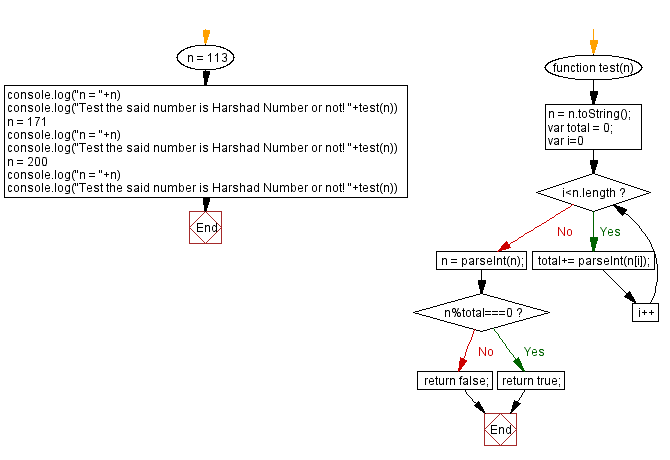# JavaScript: Test if a number is a Harshad Number or not

## JavaScript Math: Exercise-90 with Solution

Write a JavaScript program to check whether a number is a Harshad Number or not.

In recreational mathematics, a harshad number in a given number base, is an integer that is divisible by the sum of its digits when written in that base.
Example: Number 200 is a Harshad Number because the sum of digits 2 and 0 and 0 is 2 (2+0+0) and 200 is divisible by 2. Number 171 is a Harshad Number because the sum of digits 1 and 7 and 1 is 9(1+7+1) and 171 is divisible by 9.

Test Data:
(113) -> false
(171) -> true
(200) -> true

Sample Solution:

HTML Code:

``````<!DOCTYPE html>
<html>
<meta charset="utf-8">
<title>JavaScript program to Test if a number is a Harshad Number or not</title>
<body>

</body>
</html>
```
```

JavaScript Code:

``````function test(n) {
n = n.toString();
var total = 0;
for (var i=0; i<n.length; i++)
{
total+= parseInt(n[i]);
}
n = parseInt(n);
if (n%total===0)
{
return true;
}
return false;
}
n = 113
console.log("n = "+n)
console.log("Test the said number is Harshad Number or not! "+test(n))
n = 171
console.log("n = "+n)
console.log("Test the said number is Harshad Number or not! "+test(n))
n = 200
console.log("n = "+n)
console.log("Test the said number is Harshad Number or not! "+test(n))
```
```

Sample Output:

```n = 113
Test the said number is Harshad Number or not! false
n = 171
Test the said number is Harshad Number or not! true
n = 200
Test the said number is Harshad Number or not! true
```

Flowchart:Live Demo:

See the Pen javascript-math-exercise-90 by w3resource (@w3resource) on CodePen.

Improve this sample solution and post your code through Disqus

What is the difficulty level of this exercise?

Test your Programming skills with w3resource's quiz.

﻿